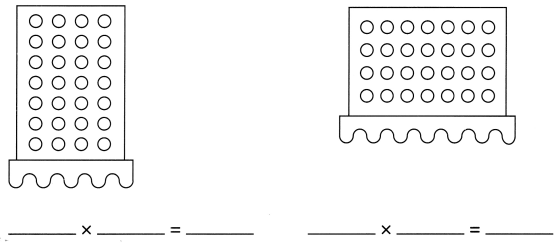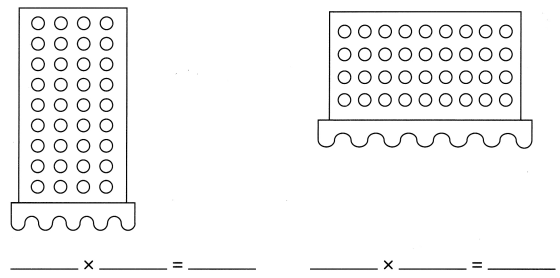# Math in Focus Grade 2 Chapter 15 Practice 4 Answer Key Multiplying 4: Using Dot Paper

This handy Math in Focus Grade 2 Workbook Answer Key Chapter 15 Practice 4 Multiplying 4: Using Dot Paper detailed solutions for the textbook questions.

## Math in Focus Grade 2 Chapter 15 Practice 4 Answer Key Multiplying 4: Using Dot Paper

Solve.

Example
There are 3 boxes of crayons. There are 4- crayons in each box. How many crayons are there in all?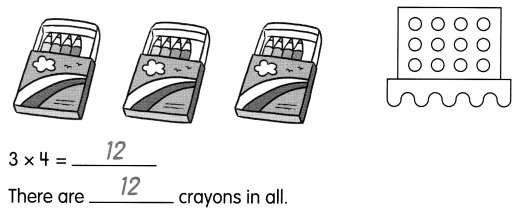Question 1.
There are 6 toy cars in a toy box. Each car has 4 wheels. How many wheels are there in all?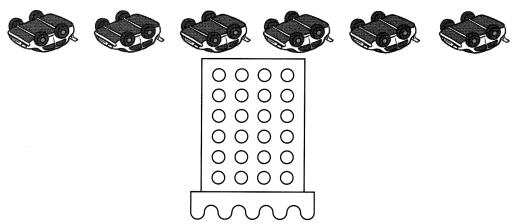___________ × 4 = __________
There are ___________ wheels in all.

6 x 4 = 24

There are 24 wheels in all.

Use dot paper to solve.

Question 2.
Mrs. Jones buys 5 T-shirts. Each T-shirt costs $4. How much does Mrs. Jones spend in all?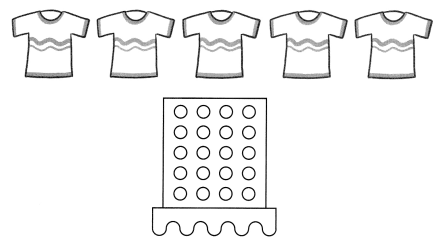___________ ×$4 = $___________ Mrs. Jones spends$___________ in all.

5 x $4 =$20

Mrs. Jones spends \$20 in all.

Question 3.
There are 8 bags. Each bag has 4 muffins. How many muffins are there in all?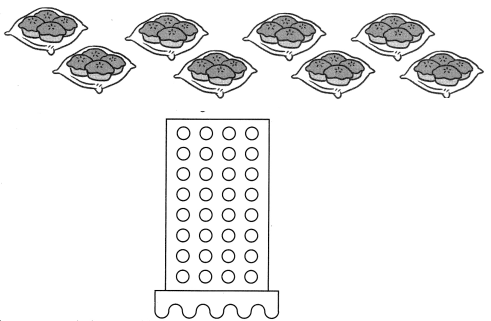___________ × ___________ = ___________
There are ___________ muffins in all.

8 x 4 = 32

There are 32 muffins in all.

Example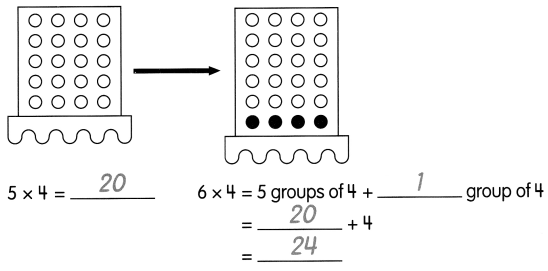Question 4.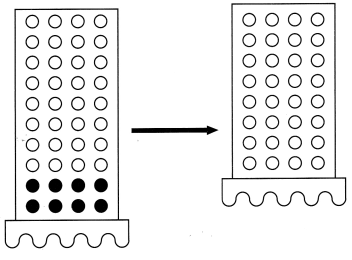10 × 4 = ___________
8 × 4 = 10 groups of 4 – ____________ groups of 4
= ____________ – 8
= ____________

10 × 4 = 40
8 × 4 = 10 groups of 4 – 2 groups of 4
= 40 – 8
= 32

Question 5.
7 × 4 = _________ + 8
= ___________

7 x 4 = ( 5 x 4 ) + 8

= 20 + 8

= 28.

Question 6.
9 × 4 = _________ – 4
= ____________

9 x 4 = ( 10 x 4 ) – 4

= 40 – 4

= 36

Example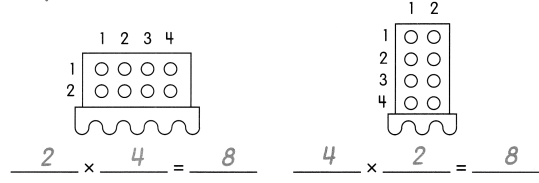Question 7.